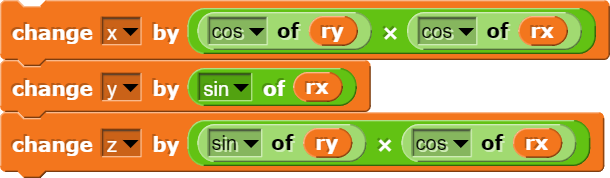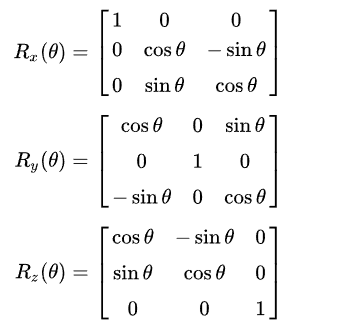# 3D movement and rotation?

I found this workaround for MOVE:
[scratchblocks]
go to x: ((x position) + (([sin v] of (direction)) * (number))) y: ((y position) + (([cos v] of (direction)) * (number)))
[/scratchblocks]
and I want to implement it for 3D. I also want to implement rotation similarly. (I'm using three.js in a replit repl.)

What do you need help with?

This will move something in a specified directionI think this should work.

I have rx, ry, and rz. All of them are rotation counterclockwise around their respective axes, in radians.

rz is just the roll axis, and would not affect the direction the camera would move.

That's only true if rx and ry are both a multiple of π radians.

but how is roll supposed to affect the forward vector if the roll axis is the forward vector?
you want the camera to move in the direction of its forward vector right?
the vector that points outwards from the front of the camera?

2 and 3. Yes.

1. If I'm pointing along the x-axis, rz rotates the camera left-right, and it's rx that's roll. But if I'm pointing along the y-axis, it's ry that's roll.

ok what which rotation order do you want? in case you don't know what rotation ordering is, it's in which order these rotation matrices are multiplied to make the object rotated:i'm assuming you want YXZ since that's what the ordering most applications use for camera rotation. if YXZ is what you want, then basically my code should work. i tested it. i did flip the signs a little bit to make it work but it's still basically my code from before.

x += -sin(ry) * cos(rx)
y += sin(rx)
z += -cos(ry) * cos(rx)


the only problem is that, if the code just simply changes rx and ry like so:

ry += userTurnY() // if up or down arrow is pressed
rx += userTurnX() // if right or left arrow is pressed


and if rz isn't 0, then rotating it is weird. but translating the camera forward works as intended. trust me, i tested it.
i'm gonna figure out how to make rotation work. i'll post it when i'm finished.

I'm not sure. I think I'm using XYZ, but I'm not sure. I don't know a lot about three.js.

I wouldn't do that. BTW I start facing along the x-axis, so it's rz that would be left-right, at least until I use roll or up/down.

I basically need to figure out the x, y, and z axes relative to the camera, and be able to convert that to absolute xyz.

so basically, you have a variable rot that is a 4x4 matrix.
to rotate it, you use this:

r = r * rotationY(turnY) * rotationX(turnX) * rotationZ(turnZ)


turnX, turnY, and turnZ are how much it should turn, from user input.
rotationX, rotationY, and rotationZ return rotation matrices given the axis and angle.
the order is YXZ

now, the vectors pointing outwards to the back (vector Z), right (vector X), and up (vector Y) of the camera are stored in the rotation matrix

X1 X2 X3 0
Y1 Y2 Y3 0
Z1 Z2 Z3 0
0  0  0  0


so if you add Z to the camera's position, it will move it backward from its orientation.
if you add X to the camera's position, it will move it to the right from its orientation.
and if you add Y to the camera's position, it will move it up from its orientation.

this makes it rotate differently compared to my previous solution, but it works well for any rz value.
three.js might have methods to do these. i don't really know, i haven't used three.js in a long time.

edit: three.js has the following methods:
new Euler(x: number, y: number, z: number, order: string)
Matrix4.makeRotationFromEuler(euler: Euler): Matrix4
Matrix4.makeRotationX(theta: number): Matrix4
Matrix4.makeRotationY(theta: number): Matrix4
Matrix4.makeRotationZ(theta: number): Matrix4
Matrix4.elements: array

well i could just see the files and fork it

well i mean... you sure that is allowed? it's an offsite meeting... technically
or did they change the rules a bit since i last frequently checked here?
i'm not sure...